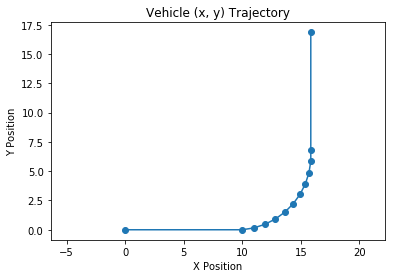# 3. Plotting Robot Motion (right angles only)

``````from matplotlib import pyplot as plt

class Vehicle:
def __init__(self):
"""
Creates new vehicle at (0,0) with a heading pointed East.
"""
self.x       = 0 # meters
self.y       = 0
self.heading = "E" # Can be "N", "S", "E", or "W"
self.history = []

# TODO-1 - Implement this function
def drive_forward(self, displacement):
"""
Updates x and y coordinates of vehicle based on
heading and appends previous (x,y) position to
history.
"""

# this line appends the current (x,y) coordinates
# to the vehicle's history. Useful for plotting
# the vehicle's trajectory. You shouldn't need to
# change this line.
self.history.append((self.x, self.y))

# vehicle currently pointing east...
self.x += displacement

# north
self.y += displacement

# west
self.x -= displacement

# south
else:
self.y -= displacement

def turn(self, direction):
if direction == "L":
self.turn_left()
elif direction == "R":
self.turn_right()
else:
print("Error. Direction must be 'L' or 'R'")
return

def turn_left(self):
"""
"""
"N" : "W",
"W" : "S",
"S" : "E",
"E" : "N",
}

# TODO-2 - implement this function
def turn_right(self):
"""
"""
"N" : "E",
"W" : "N",
"S" : "W",
"E" : "S",
}

def show_trajectory(self):
"""
Creates a scatter plot of vehicle's trajectory.
"""
X = [p for p in self.history]
Y = [p for p in self.history]

X.append(self.x)
Y.append(self.y)

plt.scatter(X,Y)
plt.plot(X,Y)
plt.show()
``````
``````# TESTING CODE 1

# instantiate vehicle
v = Vehicle()

# drive in spirals of decreasing size
v.drive_forward(8)
v.turn("L")

v.drive_forward(5)
v.turn("L")

v.drive_forward(5)
v.turn("L")

v.drive_forward(4)
v.turn("L")

v.drive_forward(4)
v.turn("L")

v.drive_forward(3)
v.turn("L")

v.drive_forward(3)
v.turn("L")

v.drive_forward(2)
v.turn("L")

v.drive_forward(2)
v.turn("L")

v.drive_forward(1)
v.turn("L")

v.drive_forward(1)

# show the trajectory. It should look like a spiral
v.show_trajectory()

# TESTING
assert(v.x == 5)
assert(v.y == 3)
first_5 = [
(0, 0),
(8, 0),
(8, 5),
(3, 5),
(3, 1)
]
assert(first_5 == v.history[:5])
print("Nice job! Your vehicle is behaving as expected!")
``````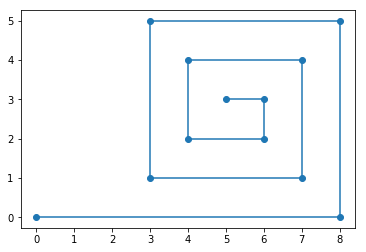Nice job! Your vehicle is behaving as expected!

``````# TESTING CODE Part 2
def test_zig_zag():
v = Vehicle()
for i in range(10):
for _ in range(4):
v.drive_forward(2)
v.turn("R")
v.drive_forward(1)
v.turn("L")
v.drive_forward(10)
v.turn("R")
first_six = [
(0,0),
(2,0),
(2,-1),
(4,-1),
(4,-2),
(6,-2)
]
v.show_trajectory()
assert(v.x == 14)
assert(v.y == -22)
assert(v.history[:6] == first_six)
print("Nice job! Your vehicle passed the zig zag test.")
test_zig_zag()
``````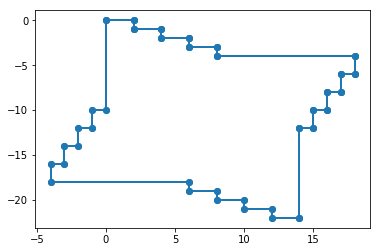Nice job! Your vehicle passed the zig zag test.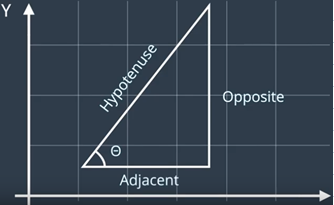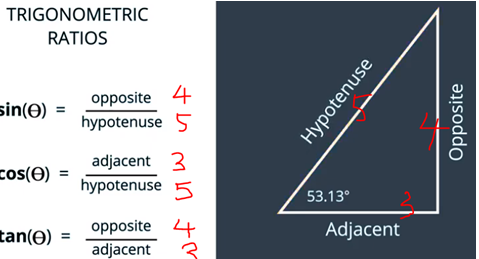# 11. Looking up Sin, Cos, and Tan

``````from math import pi
# TODO - implement this function (solution
#    code at end of notebook)

return theta*(pi/180)

for theta in [0, 30, 45, 60, 90]:
print("sin(", theta, "degrees) =", sin_theta)
``````
``````Nice work! Your degrees to radians function works!
sin( 0 degrees) = 0.0
sin( 30 degrees) = 0.49999999999999994
sin( 45 degrees) = 0.7071067811865475
sin( 60 degrees) = 0.8660254037844386
sin( 90 degrees) = 1.0
``````
``````import numpy as np
from matplotlib import pyplot as plt
def plot_sine(min_theta, max_theta):
"""
Generates a plot of sin(theta) between min_theta
and max_theta (both of which are specified in degrees).
"""
angles_degrees = np.linspace(min_theta, max_theta)
X = angles_degrees
Y = values
plt.plot(X,Y)
plt.show()

# EXERCISE 2.1 Implement this! Try not to look at the
#  implementation of plot_sine TOO much...
def plot_cosine(min_theta, max_theta):
"""
Generates a plot of sin(theta) between min_theta
and max_theta (both of which are specified in degrees).
"""
angles_degrees = np.linspace(min_theta, max_theta)
X = angles_degrees
Y = values
plt.plot(X,Y)
plt.show()
``````
``````plot_sine(0, 360)
``````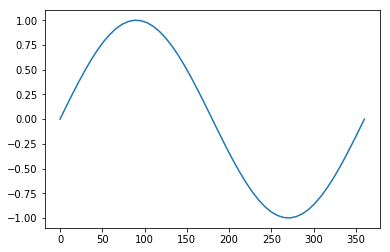``````plot_cosine(0, 360)
``````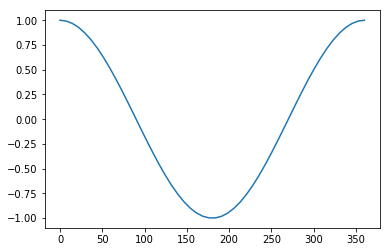# 15. Keeping Track of x and y

``````import numpy as np
from math import pi
from matplotlib import pyplot as plt

# these 2 lines just hide some warning messages.
import warnings
warnings.filterwarnings('ignore')

class Vehicle:
def __init__(self):
self.x       = 0.0 # meters
self.y       = 0.0
self.history = []

def drive_forward(self, displacement):
"""
Updates x and y coordinates of vehicle based on
heading and appends previous (x,y) position to
history.
"""

new_x = self.x + delta_x
new_y = self.y + delta_y

self.history.append((self.x, self.y))

self.x = new_x
self.y = new_y

"""
between -pi and pi.
"""

def turn(self, degrees):
rads = (degrees * pi / 180)

def show_trajectory(self):
"""
Creates a scatter plot of vehicle's trajectory.
"""
# get the x and y coordinates from vehicle's history
X = [p for p in self.history]
Y = [p for p in self.history]

# don't forget to add the CURRENT x and y
X.append(self.x)
Y.append(self.y)

# create scatter AND plot (to connect the dots)
plt.scatter(X,Y)
plt.plot(X,Y)

plt.title("Vehicle (x, y) Trajectory")
plt.xlabel("X Position")
plt.ylabel("Y Position")
plt.axes().set_aspect('equal', 'datalim')
plt.show()
``````
``````# Use this testing code to check your code for correctness.

test_drive_forward(Vehicle)
``````
``````# instantiate vehicle
v = Vehicle()

# drive forward 10 meters
v.drive_forward(10)

# turn left in 10 increments of 9 degrees each.
for _ in range(10):
v.turn(9.0)
v.drive_forward(1)

v.drive_forward(10)

v.show_trajectory()
``````